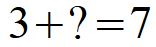Algebra basics

I've heard it said, math was just fine until they put all those letters in it.For many, algebra is the beginning of the end of their math exploration. And yet, crazy as it may sound, algebra is simply the continuation of the basic rules of math one learns the first year or two in math.

So, let's have some high-adventure with math!What's with all those letters?

The basis of all mathematics is simple addition. So take a simple example.I don't know about you, but when I was learning addition I was quizzed on my understanding of adding by being asked to fill in the blanks.Actually, this is the beginning of algebra. Algebra begins with an acknowledgment that we don't know everything.

In algebra, we apply the usual rules of mathematics while at the same time remembering that not all the numbers are known. Also in algebra, we use symbols for the unknown. Often it is of the form of a letter.Of course, we could use any symbol or, in the field of computer programming, words, or phrases.Fair is fair

The trick is to use the behavior of numbers to come up with rules that can be applied to algebra questions to find unknown numbers. The examples used here are so simple that using the full machinery of algebra is overkill – but it will help make the point.

Suppose we simply add another term onto the first math equation above.Do the math, things don't add up right. We began with a correct math statement and corrupted it into a mess. This is opposite to the idea of logic which is as follows: If you begin with a true statement, logic is the process of producing more truth out of it.

We began with a truth, but ended with an untruth. This is not logical. We've uncovered the math secret.Math is not actually about numbers. It is about logically working with information. So if we begin with a statement that is to be true, we need to restrict our rules to keep the statement true.

So in light of this, we need to keep the earlier math equation true. One way to do this is to be fair to both sides.We have our first rule of algebra: What you do on one side of the equation must be done on both. In the case of addition, this means adding to both sides. The same applies to subtraction.

Example:

Suppose we have the questionThis is the same as asking the question "what number plus five is six?" It doesn't take too much math skill to recognize the answer is one, but let's do it the algebra way anyway. To do this we eliminate the add 5 by subtracting 5 from both sides.From a logical point of view, nothing has really changed. If we accept that the value of x makes the first true, this statement must still be true (check it yourself, 1 + 5 - 5 does equal 6 – 5). But what we have gained is that the unwanted plus five can be taken out since 5 – 5 is just zero. Our statement gives:Surprise, surprise, we get the answer we expected all along.

Again, our simple example was easy enough to figure out without all the “mumbo, jumbo.” But the true power of algebra, or math in general, comes from solving complex questions that we cannot figure out on our own.

It's like magic sometimes.Opposites attract

Let's continue exploring the rules of math. Suppose that I simply moved the + 4 to the other side of the original math statement.OK, this is another problem. Thou shalt not simply move terms to the other side of an equation. But suppose that we flip the plus to a minus.Check it out, it is still correct.

We have a second rule: In math, you move something to the opposite side by making it the opposite.

Some opposite pairs are as follows:

• Addition and subtraction are opposites.

• Multiplication and division are opposites.

In the earlier algebra example, we could have just moved the + 5 over as - 5.Again, we get the same result as above.

Certainly, this is a brief introduction to the rules of algebra. Some will, no doubt, find it about as exciting as watching the grass in the lawn grow.

But there is an important life lesson here. Figuring out or learning rational rules that make sense, and are powerful tools to unlock doors that we cannot otherwise open. In this way, our potential can be reached more fully.

This is true in math, and in life.

Great films

Infinity

(1996) A film starring Matthew Broderick about the true-life love story between physicist Richard Feynman and his first wife Arline. Feynman's flair for math and physics comes through, but you don't need to be a math or science nerd to appreciate it.

Warnings: There are a couple of scenes that aren't suitable for children. Also, be forewarned, it has a tear-jerker ending.

On the Web

Basic Algebra - An Introduction

This site goes over the basic rules of algebra.

Introduction to Algebra (Algebra is great fun - you get to solve puzzles!)

More like the above.

Don't miss out on future posts! Sign up for our email list and like us on Facebook!